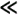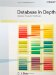# Quantification

 I showed in the previous section that one way to get a proposition from a predicate is to invoke it with an appropriate set of arguments. But there's another way, too, and that's by means of quantification. Let p(x) be a monadic predicate (I show the single parameter x explicitly, for clarity). Then: The expression: ` EXISTS x ( p ( x ) ) ` is a proposition, and it means: "There exists at least one possible argument value a corresponding to the parameter x such that p(a) evaluates to TRUE" (in other words, the argument value a satisfies predicate p). For example, if p is the predicate "x is a logician," then: ` EXISTS x ( x is a logician ) ` is a proposition one that evaluates to TRUE, as it happens (for example, take a to be Bertrand Russell). The expression: ` FORALL x ( p ( x ) ) ` is a proposition, and it means: "All possible argument values a corresponding to the parameter x are such that p(a) evaluates to TRUE" (in other words, all such argument values a satisfy predicate p). For example, if again p is the predicate "x is a logician," then: ` FORALL x ( x is a logician ) ` is a proposition one that evaluates to FALSE, as it happens (for example, take a to be George W. Bush). Observe that it's sufficient to produce a single example to show the truth of the EXISTS proposition and a single counterexample to show the falsity of the FORALL proposition. Observe too in both cases that the parameter must be constrained to "range over" some set of permissible values (the set of all people, in the example). I'll come back to this point later, in the section "Database Constraints." The term used in logic for the expressions EXISTS x and FORALL x in the foregoing discussion is quantifiers (the term derives from the verb to quantify, which simply means to express as a quantity that is, to say how much of something there is or how many somethings there are). Quantifiers of the form EXISTS...are said to be existential; quantifiers of the form FORALL...are said to be universal. (And in logic texts, EXISTS is usually represented by a backward E and FORALL by an upside-down A. I use the keywords EXISTS and FORALL for typographical reasons not to mention readability.) By way of another example, let q be the dyadic predicate "x is taller than y." If we "quantify existentially over x," we obtain: ` EXISTS x ( x is taller than y ) ` Now, this statement is not a proposition, because it isn't unequivocally either true or false; in fact, it's a monadic predicate it has a single parameter, y. Suppose we invoke this predicate with argument Steve. We obtain: ` EXISTS x ( x is taller than Steve )` This statement is a proposition (and if there exists at least one person Arnold, say who's taller than Steve, then it evaluates to TRUE, of course). But another way to obtain a proposition from the original predicate q is to quantify over both of the parameters x and y. For example: ` EXISTS x ( EXISTS y ( x is taller than y ) ) ` This statement is indeed a proposition; it evaluates to FALSE only if nobody is taller than anybody else and to TRUE otherwise (think about it!). There are several lessons to be learned from this example: To obtain a proposition from an n-adic predicate by quantification, it's necessary to quantify over every parameter. More generally, if we quantify over m parameters (mk-adic predicate, where k = n-m.Let's focus on existential quantification only for the moment. Then there are apparently two different propositions we can obtain in the example by "quantifying over everything":` EXISTS x ( EXISTS y ( x is taller than y) ) EXISTS y ( EXISTS x ( x is taller than y) ) ` It should be clear, however, that these two propositions both mean the same thing: "There exist two persons x and y such that x is taller than y." More generally, in fact, it's easy to see that a series of like quantifiers (all existential or all universal) can be written in any sequence we choose without changing the overall meaning of the predicate or proposition in question. We therefore allow unnecessary parentheses to be dropped, as here:` EXISTS y EXISTS x ( x is taller than y ) ` By contrast, with unlike quantifiers, the sequence matters, in general (see the next point).Of course, we can use either EXISTS or FORALL in each of the necessary quantifiers when "quantifying over everything." In the following example, therefore, there are six distinct propositions that can be obtained by fully quantifying, and I've listed them below. (Actually there are eight, but two of those eight can be eliminated by virtue of the previous point.) I've also shown a precise natural-language interpretation in each case. Note that those interpretations are all logically different! Please note too, however, that I've had to assume in connection with those interpretations that there do exist at least two people "in the universe," as it were. I'll come back to this assumption later (in a discussion of empty ranges in the section "More on Quantification").` EXISTS x ( EXISTS y ( x is taller than y ) ) ` Meaning: Somebody is taller than somebody else; TRUE, unless everybody is the same height. ` EXISTS x ( FORALL y ( x is taller than y ) ) ` Meaning: Somebody is taller than everybody (that particular somebody included!); clearly FALSE. ` FORALL x ( EXISTS y ( x is taller than y ) ) ` Meaning: Everybody is taller than somebody; clearly FALSE. ` EXISTS y ( FORALL x ( x is taller than y ) ) ` Meaning: Somebody is shorter than everybody (that particular somebody included!); clearly FALSE. ` FORALL y ( EXISTS x ( x is taller than y ) ) ` Meaning: Everybody is shorter than somebody; clearly FALSE.` FORALL x ( FORALL y ( x is taller than y ) ) ` Meaning: Everybody is taller than everybody; clearly FALSE. Finally (at the risk of belaboring the obvious), even though five out of six of the foregoing propositions all evaluate to the same truth value, FALSE, it doesn't follow that they all mean the same thing, and indeed they don't; in fact, no two of them do.Database in Depth: Relational Theory for Practitioners
ISBN: 0596100124
EAN: 2147483647
Year: 2006
Pages: 127
Authors: C.J. Date

Similar book on Amazon